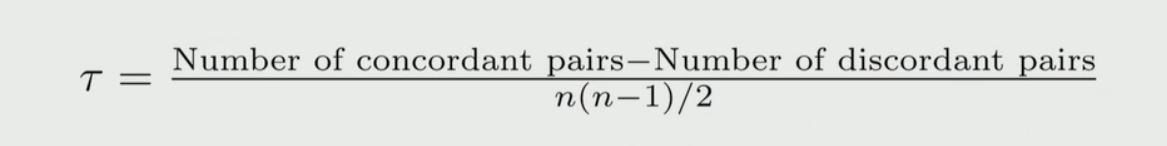# Python – Kendall Rank Correlation Coefficient

What is correlation test?
The strength of the association between two variables is known as correlation test. For instance, if we are interested to know whether there is a relationship between the heights of fathers and sons, a correlation coefficient can be calculated to answer this question.

Methods for correlation analysis:
There are mainly two types of correlation:

• Parametric Correlation – Pearson correlation(r) : It measures a linear dependence between two variables (x and y) is known as a parametric correlation test because it depends on the distribution of the data.
• Non-Parametric Correlation – Kendall(tau) and Spearman(rho): They are rank-based correlation coefficients, are known as non-parametric correlation.

Kendall Rank Correlation Coefficient formula:where,

• Concordant Pair: A pair of observations (x1, y1) and (x2, y2) that follows the property
• x1 > x2 and y1 > y2 or
• x1 < x2 and y1 < y2
• Discordant Pair: A pair of observations (x1, y1) and (x2, y2) that follows the property
• x1 > x2 and y1 < y2 or
• x1 < x2 and y1 > y2
• n: Total number of samples

Note: The pair for which x1 = x2 and y1 = y2 are not classified as concordant or discordant and are ignored.

Example: Let’s consider two experts ranking on food items in the below table.

Items Expert 1 Expert 2
1 1 1
2 2 3
3 3 6
4 4 2
5 5 7
6 6 4
7 7 5

The table says that for item-1, expert-1 gives rank-1 whereas expert-2 gives also rank-1. Similarly for item-2, expert-1 gives rank-2 whereas expert-2 gives rank-3 and so on.

Step1:
At first, according to the formula, we have to find the number of concordant pairs and the number of discordant pairs. So take a look at item-1 and item-2 rows. Let for expert-1, x1 = 1 and x2 = 2. Similarly for expert-2, y1 = 1 and y2 = 3. So the condition x1 < x2 and y1 < y2 satisfies and we can say item-1 and item-2 rows are concordant pairs.

Similarly take a look at item-2 and item-4 rows. Let for expert-1, x1 = 2 and x2 = 4. Similarly for expert-2, y1 = 3 and y2 = 2. So the condition x1 < x2 and y1 > y2 satisfies and we can say item-2 and item-4 rows are discordant pairs.

Like that, by comparing each row you can calculate the number of concordant and discordant pairs. The complete solution is given in the below table.

1
2 C
3 C C
4 C D D
5 C C C C
6 C C C D D
7 C C C C D D
1 2 3 4 5 6 7

Step 2:
So from the above table, we found that,
The number of concordant pairs is: 15
The number of discordant pairs is: 6
The total number of samples/items is: 7

Hence by applying the Kendall Rank Correlation Coefficient formula

tau = (15 – 6) / 21 = 0.42857

This result says that if it’s basically high then there is a broad agreement between the two experts. Otherwise, if the expert-1 completely disagrees with expert-2 you might get even negative values.

kendalltau() : Python functions to compute Kendall Rank Correlation Coefficient in Python

Syntax:
kendalltau(x, y)

• x, y: Numeric lists with the same length

Code: Python program to illustrate Kendall Rank correlation

## Python

 `# Import required libraries ` `from` `scipy.stats ``import` `kendalltau ` ` `  `# Taking values from the above example in Lists ` `X ``=` `[``1``, ``2``, ``3``, ``4``, ``5``, ``6``, ``7``] ` `Y ``=` `[``1``, ``3``, ``6``, ``2``, ``7``, ``4``, ``5``] ` ` `  `# Calculating Kendall Rank correlation ` `corr, _ ``=` `kendalltau(X, Y) ` `print``(``'Kendall Rank correlation: %.5f'` `%` `corr) ` ` `  `# This code is contributed by Amiya Rout `

Output:

`Kendall Rank correlation: 0.42857`

My Personal Notes arrow_drop_upCheck out this Author's contributed articles.

If you like GeeksforGeeks and would like to contribute, you can also write an article using contribute.geeksforgeeks.org or mail your article to contribute@geeksforgeeks.org. See your article appearing on the GeeksforGeeks main page and help other Geeks.

Please Improve this article if you find anything incorrect by clicking on the "Improve Article" button below.

Article Tags :

Be the First to upvote.

Please write to us at contribute@geeksforgeeks.org to report any issue with the above content.